Anzeige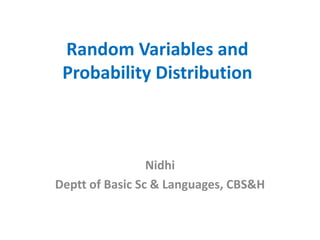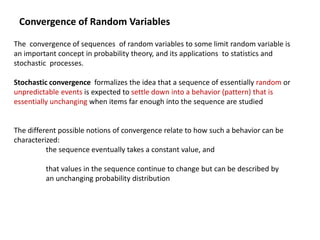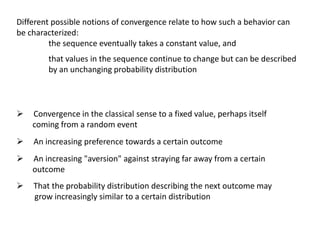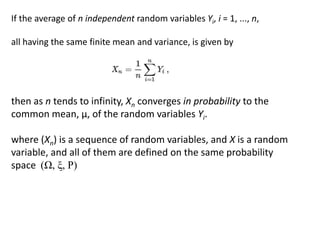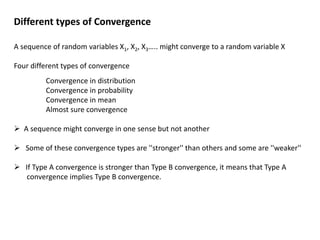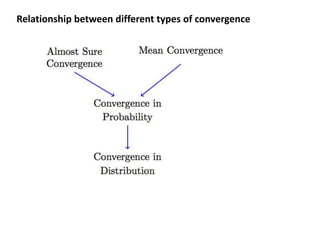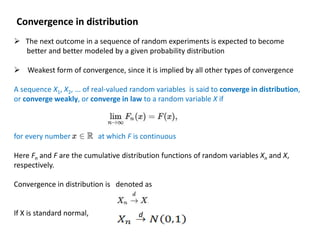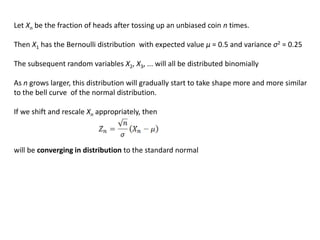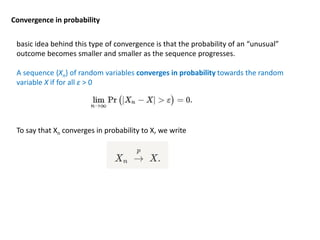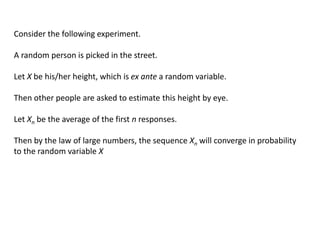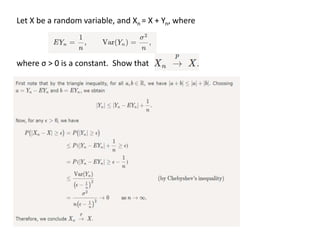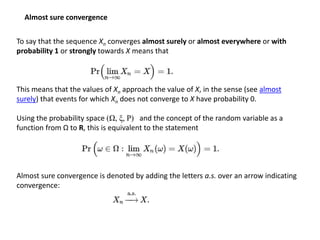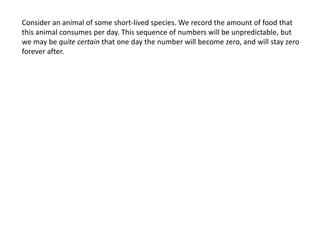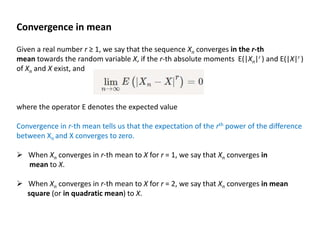1 von 14
Anzeige

### Statistical convergence.pptx

1. Random Variables and Probability Distribution Nidhi Deptt of Basic Sc & Languages, CBS&H
2. Convergence of Random Variables The convergence of sequences of random variables to some limit random variable is an important concept in probability theory, and its applications to statistics and stochastic processes. Stochastic convergence formalizes the idea that a sequence of essentially random or unpredictable events is expected to settle down into a behavior (pattern) that is essentially unchanging when items far enough into the sequence are studied The different possible notions of convergence relate to how such a behavior can be characterized: the sequence eventually takes a constant value, and that values in the sequence continue to change but can be described by an unchanging probability distribution
3.  Convergence in the classical sense to a fixed value, perhaps itself coming from a random event  An increasing preference towards a certain outcome  An increasing "aversion" against straying far away from a certain outcome  That the probability distribution describing the next outcome may grow increasingly similar to a certain distribution Different possible notions of convergence relate to how such a behavior can be characterized: the sequence eventually takes a constant value, and that values in the sequence continue to change but can be described by an unchanging probability distribution
4. If the average of n independent random variables Yi, i = 1, ..., n, all having the same finite mean and variance, is given by then as n tends to infinity, Xn converges in probability to the common mean, μ, of the random variables Yi. where (Xn) is a sequence of random variables, and X is a random variable, and all of them are defined on the same probability space (Ω, ξ, P)
5. Different types of Convergence A sequence of random variables X1, X2, X3….. might converge to a random variable X Four different types of convergence Convergence in distribution Convergence in probability Convergence in mean Almost sure convergence  A sequence might converge in one sense but not another  Some of these convergence types are ''stronger'' than others and some are ''weaker''  If Type A convergence is stronger than Type B convergence, it means that Type A convergence implies Type B convergence.
6. Relationship between different types of convergence
7. Convergence in distribution  The next outcome in a sequence of random experiments is expected to become better and better modeled by a given probability distribution  Weakest form of convergence, since it is implied by all other types of convergence A sequence X1, X2, ... of real-valued random variables is said to converge in distribution, or converge weakly, or converge in law to a random variable X if for every number at which F is continuous Here Fn and F are the cumulative distribution functions of random variables Xn and X, respectively. Convergence in distribution is denoted as If X is standard normal, d
8. Let Xn be the fraction of heads after tossing up an unbiased coin n times. Then X1 has the Bernoulli distribution with expected value μ = 0.5 and variance σ2 = 0.25 The subsequent random variables X2, X3, ... will all be distributed binomially As n grows larger, this distribution will gradually start to take shape more and more similar to the bell curve of the normal distribution. If we shift and rescale Xn appropriately, then will be converging in distribution to the standard normal
9. Convergence in probability basic idea behind this type of convergence is that the probability of an “unusual” outcome becomes smaller and smaller as the sequence progresses. A sequence {Xn} of random variables converges in probability towards the random variable X if for all ε > 0 To say that Xn converges in probability to X, we write
10. Consider the following experiment. A random person is picked in the street. Let X be his/her height, which is ex ante a random variable. Then other people are asked to estimate this height by eye. Let Xn be the average of the first n responses. Then by the law of large numbers, the sequence Xn will converge in probability to the random variable X
11. Let X be a random variable, and Xn = X + Yn, where where σ > 0 is a constant. Show that
12. Almost sure convergence To say that the sequence Xn converges almost surely or almost everywhere or with probability 1 or strongly towards X means that This means that the values of Xn approach the value of X, in the sense (see almost surely) that events for which Xn does not converge to X have probability 0. Using the probability space (Ω, ξ, P) and the concept of the random variable as a function from Ω to R, this is equivalent to the statement Almost sure convergence is denoted by adding the letters a.s. over an arrow indicating convergence:
13. Consider an animal of some short-lived species. We record the amount of food that this animal consumes per day. This sequence of numbers will be unpredictable, but we may be quite certain that one day the number will become zero, and will stay zero forever after.
14. Given a real number r ≥ 1, we say that the sequence Xn converges in the r-th mean towards the random variable X, if the r-th absolute moments E(|Xn|r ) and E(|X|r ) of Xn and X exist, and where the operator E denotes the expected value Convergence in r-th mean tells us that the expectation of the rth power of the difference between Xn and X converges to zero.  When Xn converges in r-th mean to X for r = 1, we say that Xn converges in mean to X.  When Xn converges in r-th mean to X for r = 2, we say that Xn converges in mean square (or in quadratic mean) to X. Convergence in mean
Anzeige